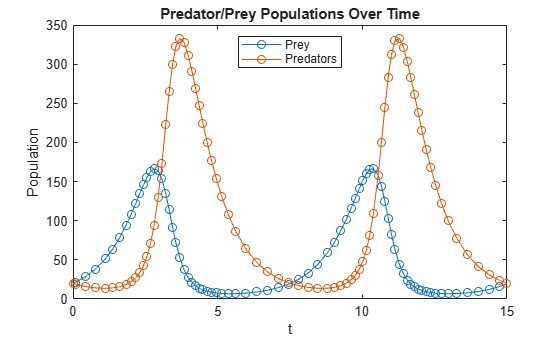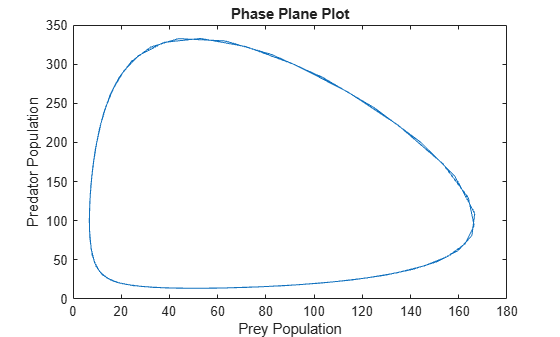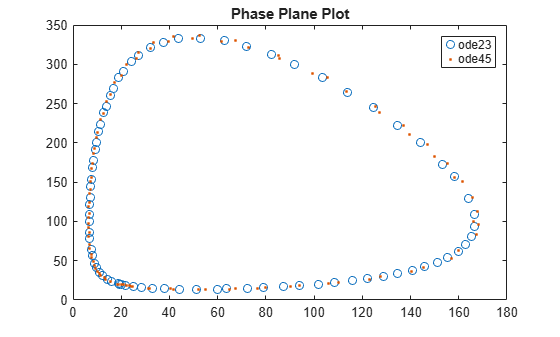Documentation

## Solve Predator-Prey Equations

This example shows how to solve a differential equation representing a predator/prey model using both `ode23` and `ode45`. These functions are for the numerical solution of ordinary differential equations using variable step size Runge-Kutta integration methods. `ode23` uses a simple 2nd and 3rd order pair of formulas for medium accuracy and `ode45` uses a 4th and 5th order pair for higher accuracy.

Consider the pair of first-order ordinary differential equations known as the Lotka-Volterra equations, or predator-prey model:

`$\begin{array}{l}\frac{\mathrm{dx}}{\mathrm{dt}}=\mathit{x}-\alpha \mathrm{xy}\\ \frac{\mathrm{dy}}{\mathrm{dt}}=-\mathit{y}+\beta \mathrm{xy}.\end{array}$`

The variables $\mathit{x}$ and $\mathit{y}$ measure the sizes of the prey and predator populations, respectively. The quadratic cross term accounts for the interactions between the species. The prey population increases when no predators are present, and the predator population decreases when prey are scarce.

### Code Equations

To simulate the system, create a function that returns a column vector of state derivatives, given state and time values. The two variables $\mathit{x}$ and $\mathit{y}$ can be represented in MATLAB as the first two values in a vector `y`. Similarly, the derivatives are the first two values in a vector `yp`. The function must accept values for `t` and `y` and return the values produced by the equations in `yp`.

` yp(1) = (1 - alpha*y(2))*y(1)`

` yp(2) = (-1 + beta*y(1))*y(2)`

In this example, the equations are contained in a file called `lotka.m`. This file uses parameter values of $\alpha =0.01$ and $\beta =0.02$.

`type lotka`
```function yp = lotka(t,y) %LOTKA Lotka-Volterra predator-prey model. % Copyright 1984-2014 The MathWorks, Inc. yp = diag([1 - .01*y(2), -1 + .02*y(1)])*y; ```

### Simulate System

Use `ode23` to solve the differential equation defined in `lotka` over the interval $0. Use an initial condition of $\mathit{x}\left(0\right)=\mathit{y}\left(0\right)=20$ so that the populations of predators and prey are equal.

```t0 = 0; tfinal = 15; y0 = [20; 20]; [t,y] = ode23(@lotka,[t0 tfinal],y0);```

### Plot Results

Plot the resulting populations against time.

```plot(t,y) title('Predator/Prey Populations Over Time') xlabel('t') ylabel('Population') legend('Prey','Predators','Location','North')```Now plot the populations against each other. The resulting phase plane plot makes the cyclic relationship between the populations very clear.

```plot(y(:,1),y(:,2)) title('Phase Plane Plot') xlabel('Prey Population') ylabel('Predator Population')```### Compare Results of Different Solvers

Solve the system a second time using `ode45`, instead of `ode23`. The `ode45` solver takes longer for each step, but it also takes larger steps. Nevertheless, the output of `ode45` is smooth because by default the solver uses a continuous extension formula to produce output at four equally spaced time points in the span of each step taken. (You can adjust the number of points with the `'Refine'` option.) Plot both solutions for comparison.

```[T,Y] = ode45(@lotka,[t0 tfinal],y0); plot(y(:,1),y(:,2),'-',Y(:,1),Y(:,2),'-'); title('Phase Plane Plot') legend('ode23','ode45')```The results show that solving differential equations using different numerical methods can produce slightly different answers.